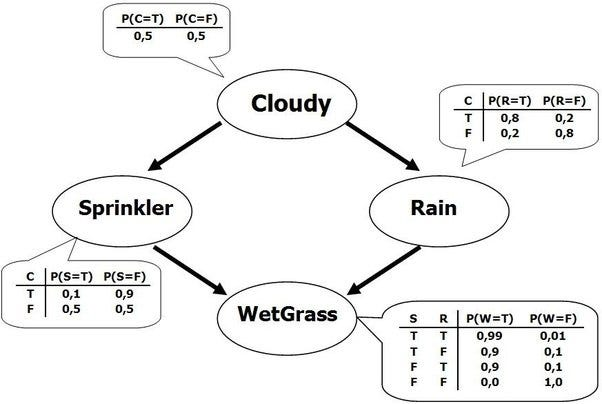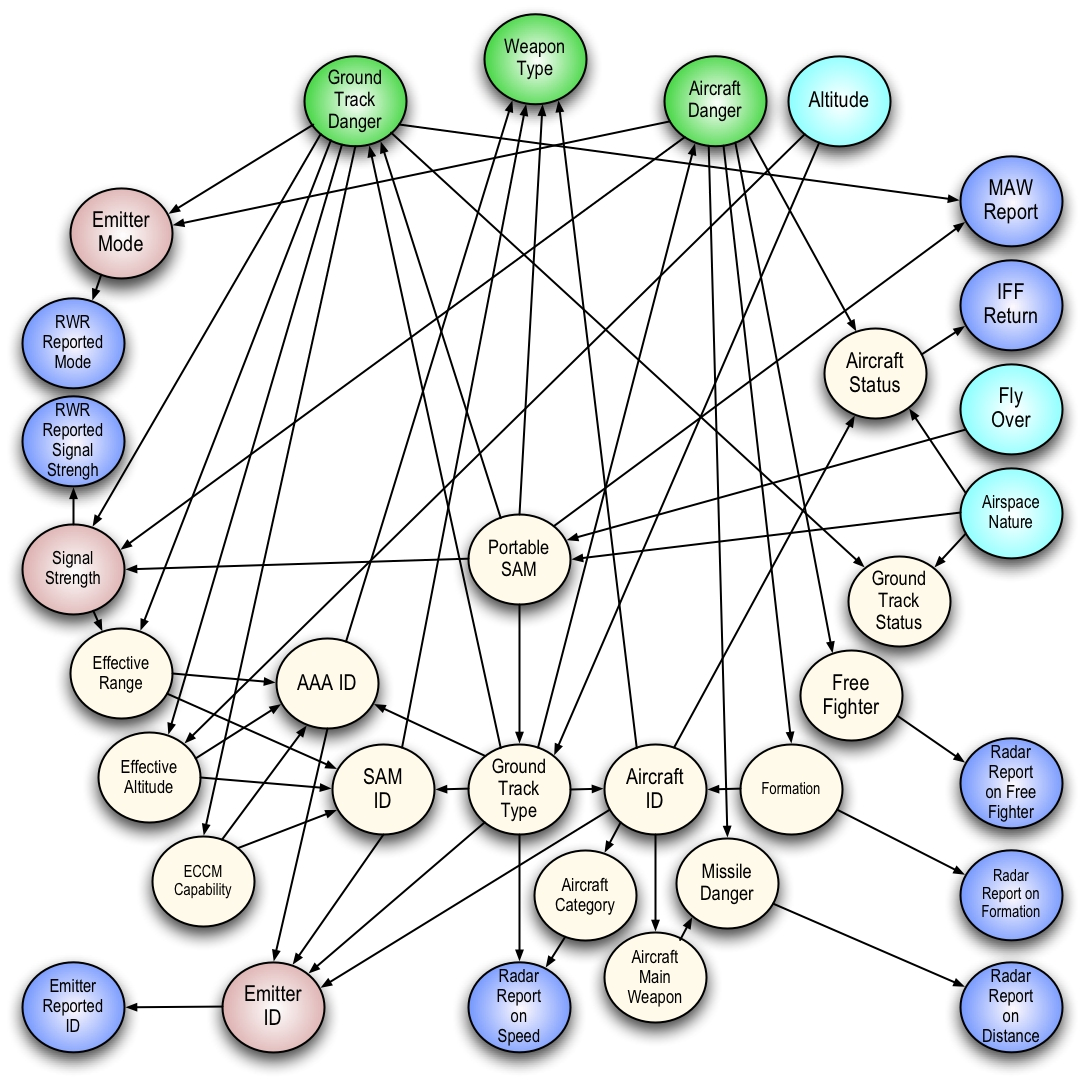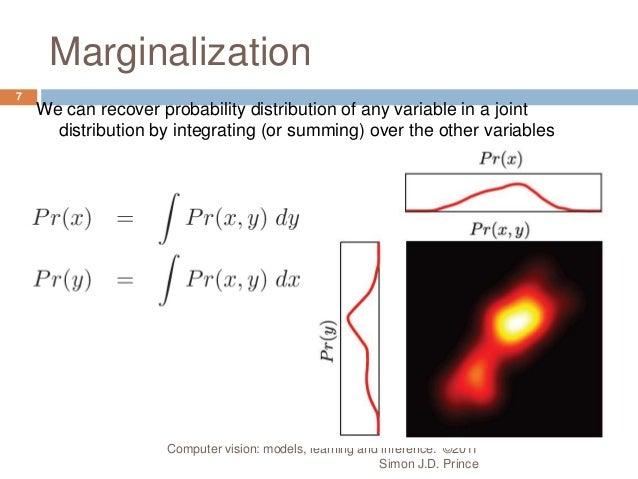# Supervised Learning: Bayesian Inference

This is the 11th in a series of class notes as I go through the Georgia Tech/Udacity Machine Learning course. The class textbook is Machine Learning by Tom Mitchell.

This chapter builds on the previous one on Bayesian Learning, and is skimpy because we skipped a lot of basic probability content.

This is also the end of a miniseries on Supervised Learning, the 1st of 3 sub disciplines within Machine Learning.

## What is Bayesian Inference?

Representing probabilities, and calculating them. For example, what is the probability of X happening given Y? But on steroids.

## Bayesian Networks

Also known as Belief Networks or Graphical Models.

The idea is to represent conditional relationships as nodes on a directed acyclic graph. Edges are therefore dependencies to be considered in your model.This can look like a neural net. Dependencies can skip levels and therefore the network’s connections can grow exponentially with every variable:This makes inference in complex Bayesian networks hard to do.

One thing to note about dependencies is that they don’t necessarily reflect cause-and-effect relationships, just ones that are conditionally dependent on the other. Perhaps more importantly, lack of dependencies are very good, because they reflect conditional independence.

The acyclic nature of belief networks mean you can do a topological sort of the nodes to order your calculations.

## Inferencing Rules

Three handy rules we use in Bayesian Inference are:

• Marginalization (intuitively, adding up the conditional probabilities)• Chain rule (joint distribution of two attributes is an attribute 1’s probability, times probability of other attributes given attribute 1)
• Bayes rule (re-expressing conditional probabilities)## Naive Bayes

Naive Bayes is a special case of Bayesian Networks, that assumes ALL attributes are conditionally independent of each other, i.e. a very very simple network with just one layer:If you take the top node as a class, and take all the child nodes as attributes, you can reverse the direction of the Bayes Net and infer the class from the attributes, arriving at a Naive Bayes Classifier:## Naive Bayes: Pros and Cons

There are a number of benefits to this approach:

• It makes inference (normally a np-hard problem) cheap
• It is linear, not exponential, in number of attributes
• It is easy to estimate these parameters with labeled data (by simple count)
• connects inference and classification - instead of only generating probabilities of attributes, you can flip it and generate classification
• Empirically it is very successful - Google has a patented version of Naive Bayes used for spam filtering.

However:

• its “naïveté” comes from assuming that there are no interrelationships between any of the attributes, which is hard to believe
• the answer is: yes, its inaccurate, but like we said in the last lesson we don’t care about getting the exact right hypothesis to estimate probabilities, we just care about getting the right answer in classification. So you just need to be directionally correct.
• Relying on an empirical count means missing attributes have zero estimated probability
• this exposes you to inductive bias and overfitting to your data
• yes, this is a problem, so in practice people “smooth probabilities” by initializing them with a small nonzero weight.

## Next in our series

Further notes on this topic:

Hopefully that was a good introduction to Bayesian Inference. I am planning more primers and would love your feedback and questions on:

• Overview
• Supervised Learning
• Unsupervised Learning
• Reinforcement Learning
• Markov Decision Processes - week of Mar 25
• “True” RL - week of Apr 1
• Game Theory - week of Apr 15

Tagged in:

Leave a reaction if you liked this post! 🧡